## UI Custom

Contains a collection of UI fields which can be added to a card on the MetricMonkey Custom tab.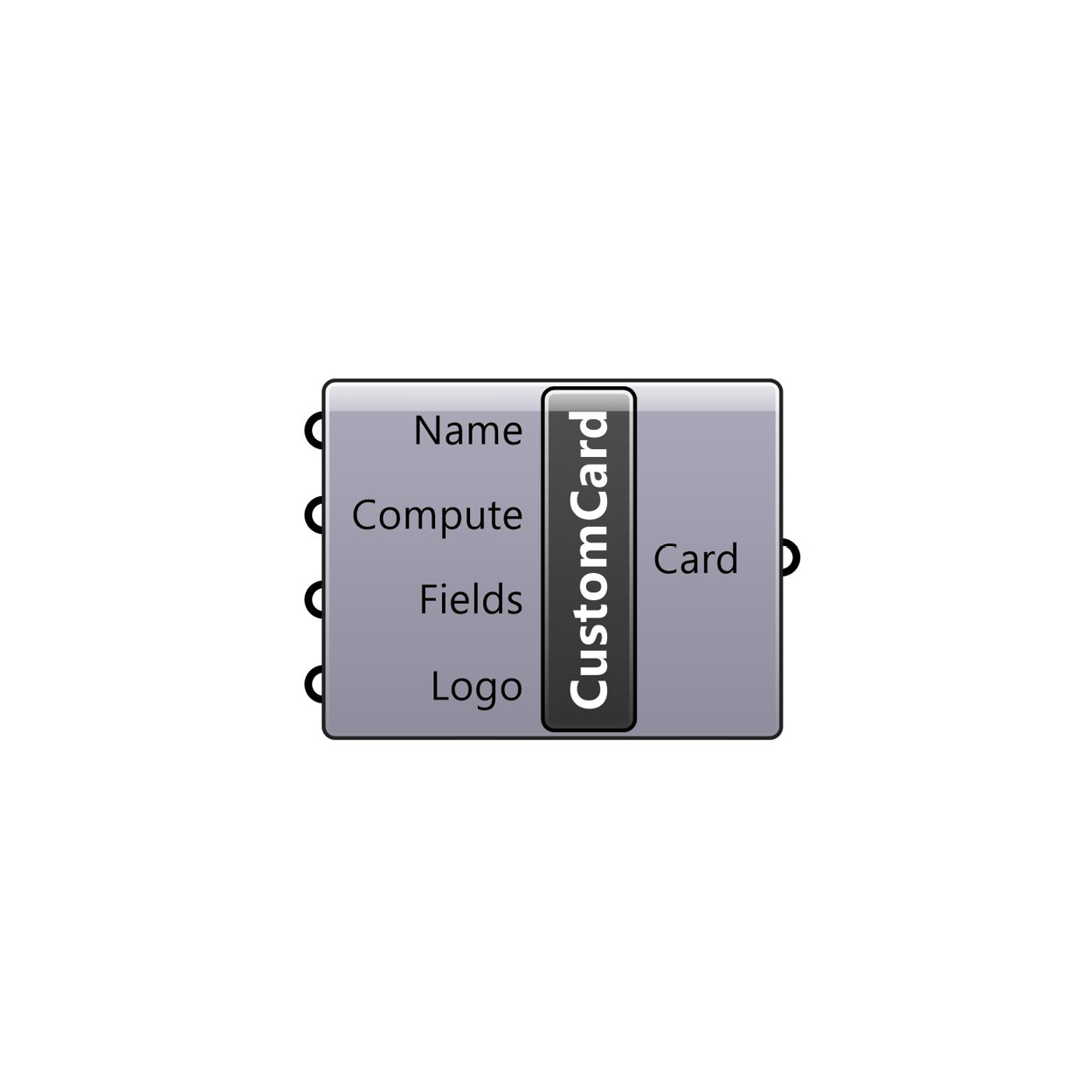### Custom Card

Creates a MetricMonkey Custom Card. Use in conjunction with the Launch Window component.

Input: Name (Text), Compute (Boolean), Fields (Varies), and Logo (File Path)

Output: Card (MetricMonkey Card).### Text Box

Creates a MetricMonkey Text Box field. Use in conjunction with the Custom Card component.

Input: Label (Text), Default Text (Text), Data Type (Integer).

Output: TextBox (MetricMonkey TextBox).### Combo Box

Creates a MetricMonkey Combo Box field. Use in conjunction with the Custom Card component.

Input: Label (Text), Default List (Data), Default Index (Integer).

Output: ComboBox (MetricMonkey ComboBox).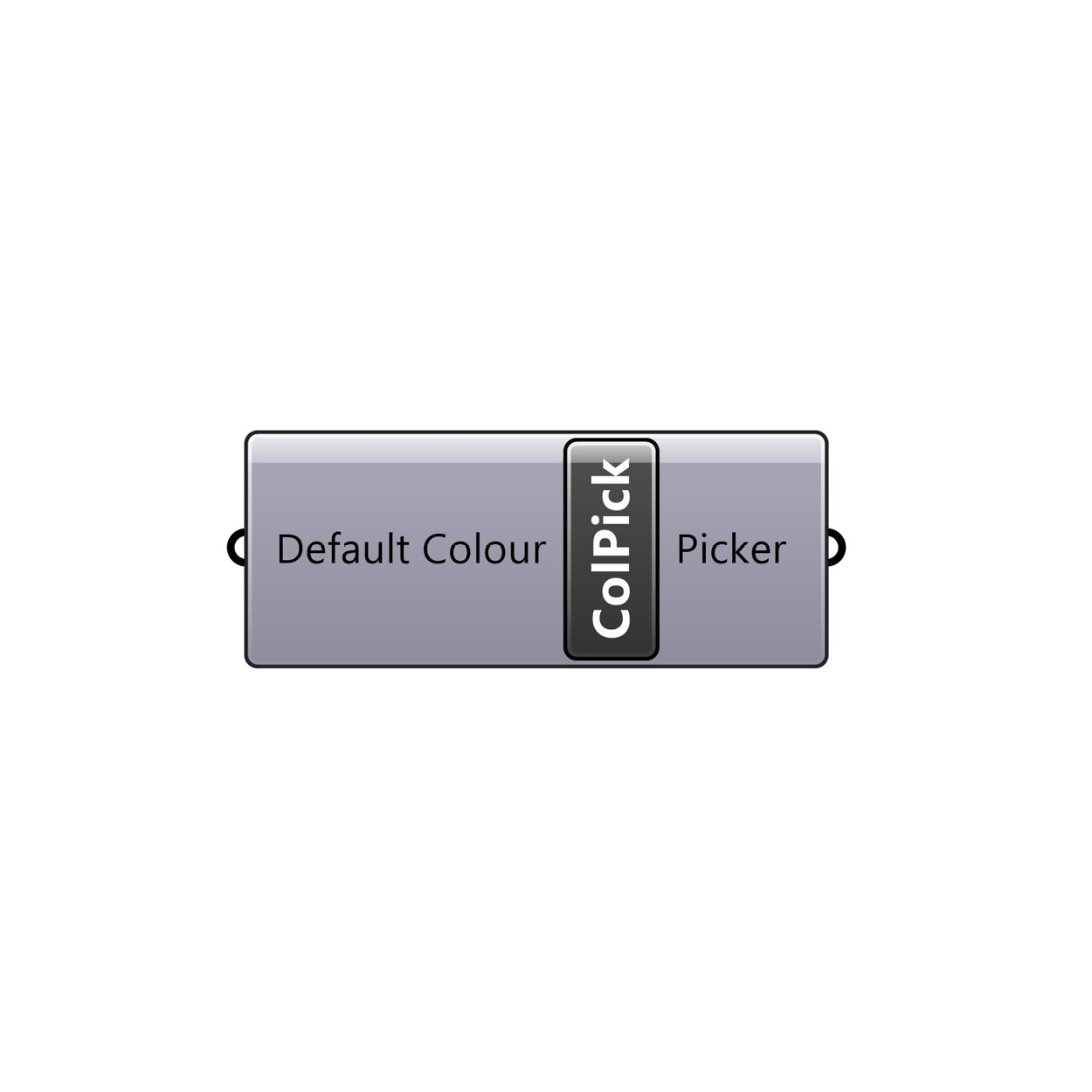### Colour Picker

Creates a MetricMonkey Colour Picker field. Use in conjunction with the Custom Card component.

Input: Default Colour (Colour).

Output: Picker (MetricMonkey ColourPicker).

## Create

Contains a collection of components to create MetricMonkey classes.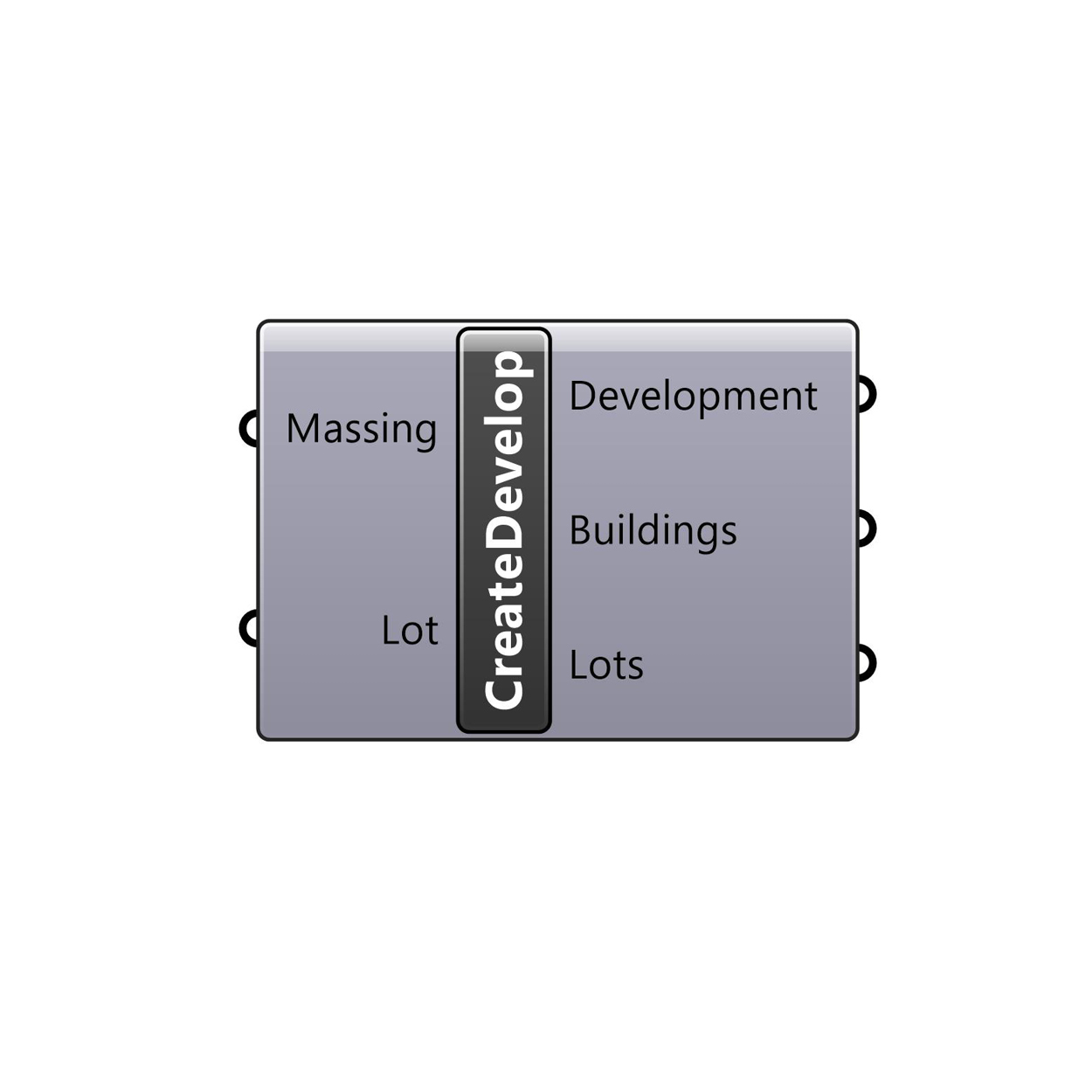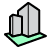### Create Development

Creates a MetricMonkey Development from MetricMonkey Masses and Lots.

Inputs: Massing (MetricMonkey Mass), and Lot (MetricMonkey Lot).

Outputs: Development (MetricMonkey Development), Buildings (MetricMonkey Building), and Lots (MetricMonkey Lot).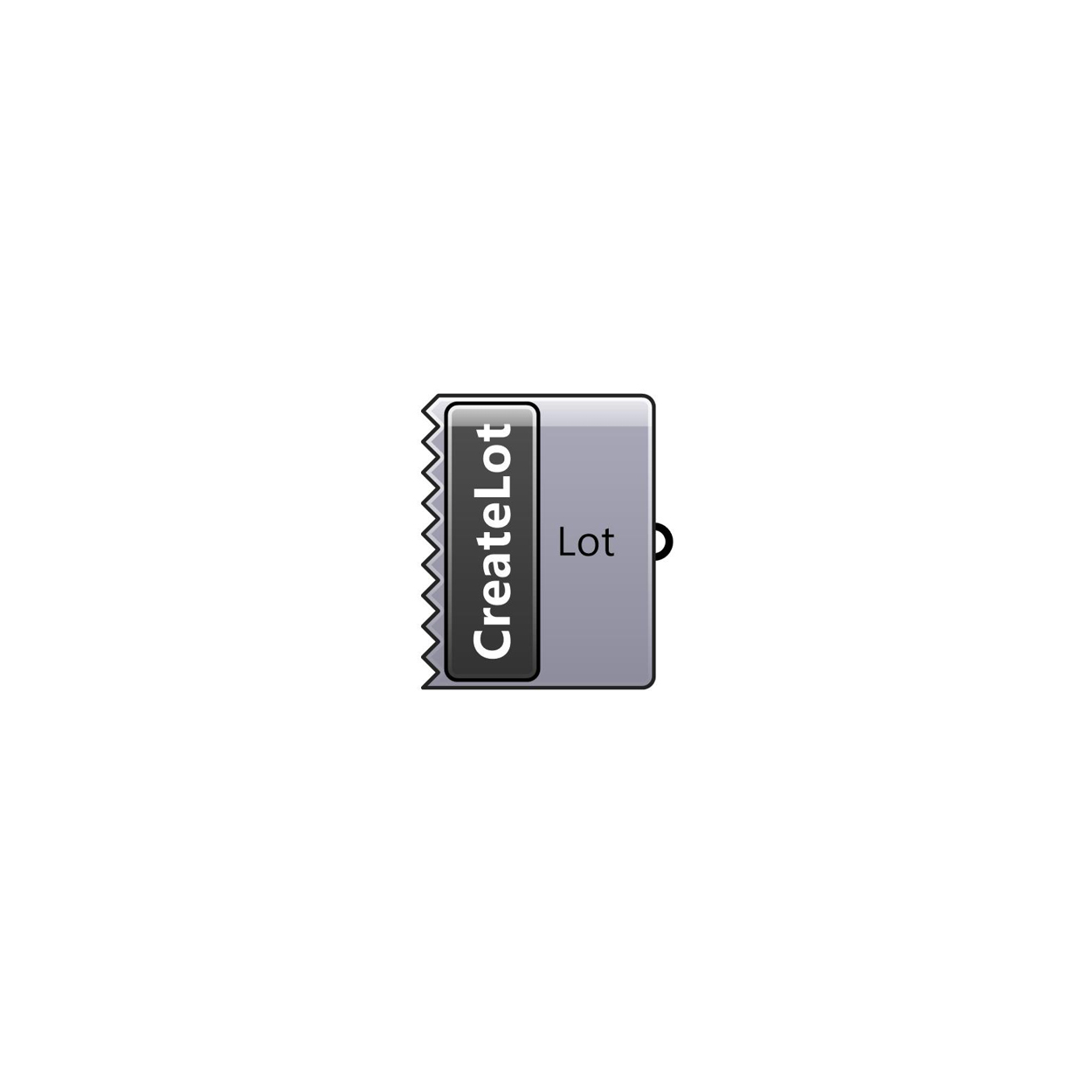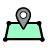### Create Lot

Creates a MetricMonkey Lot given a closed Rhino polycurve. Polycurve is referenced from the Layer defined in the Rhino Layers card.

Input: N/A

Output: Lot (MetricMonkey Lot).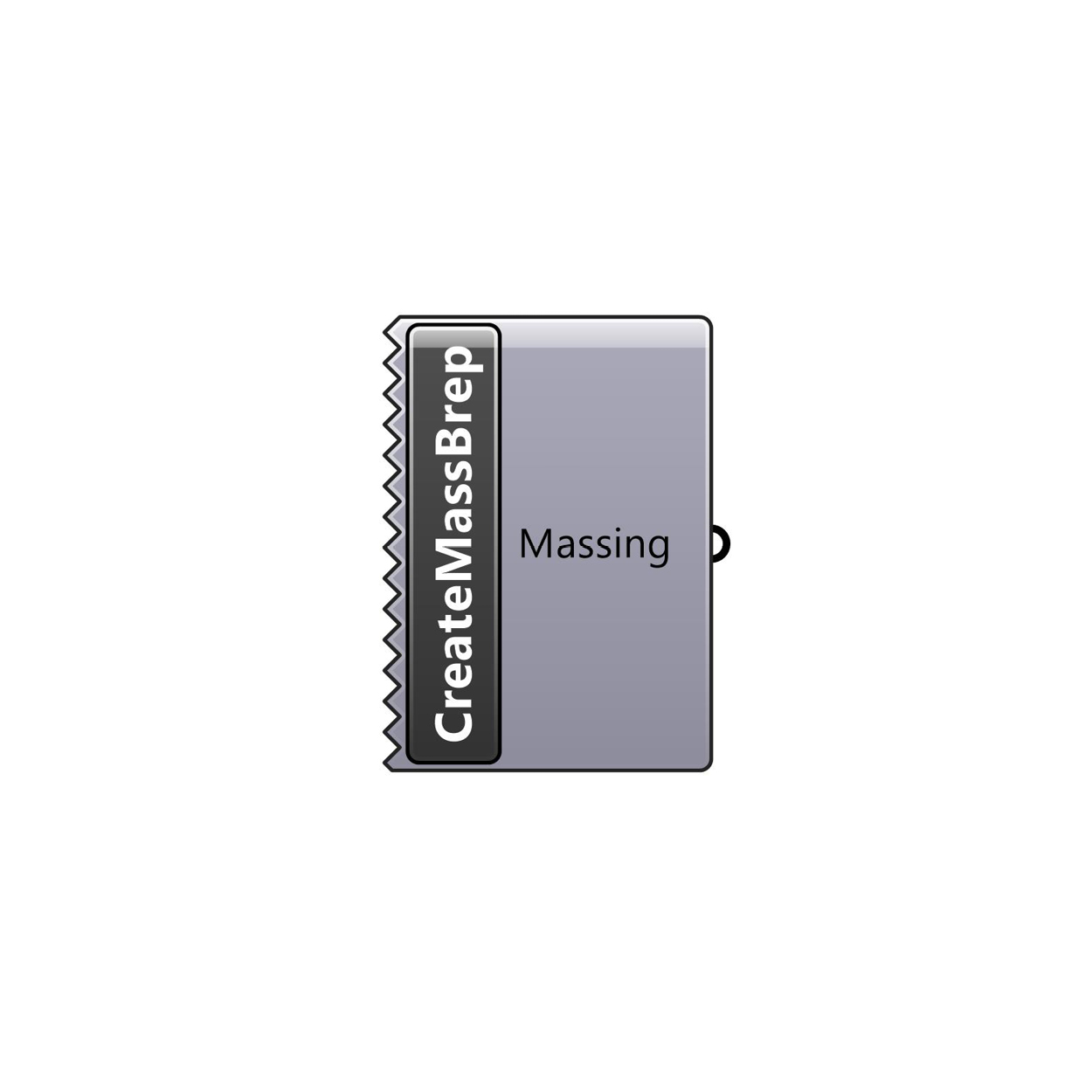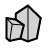### Create Massing from Brep

Creates a MetricMonkey Massing from a closed Rhino brep. Brep is referenced from the Layer defined in the Rhino Layers card.

Input: N/A

Output: Massing (MetricMonkey Mass).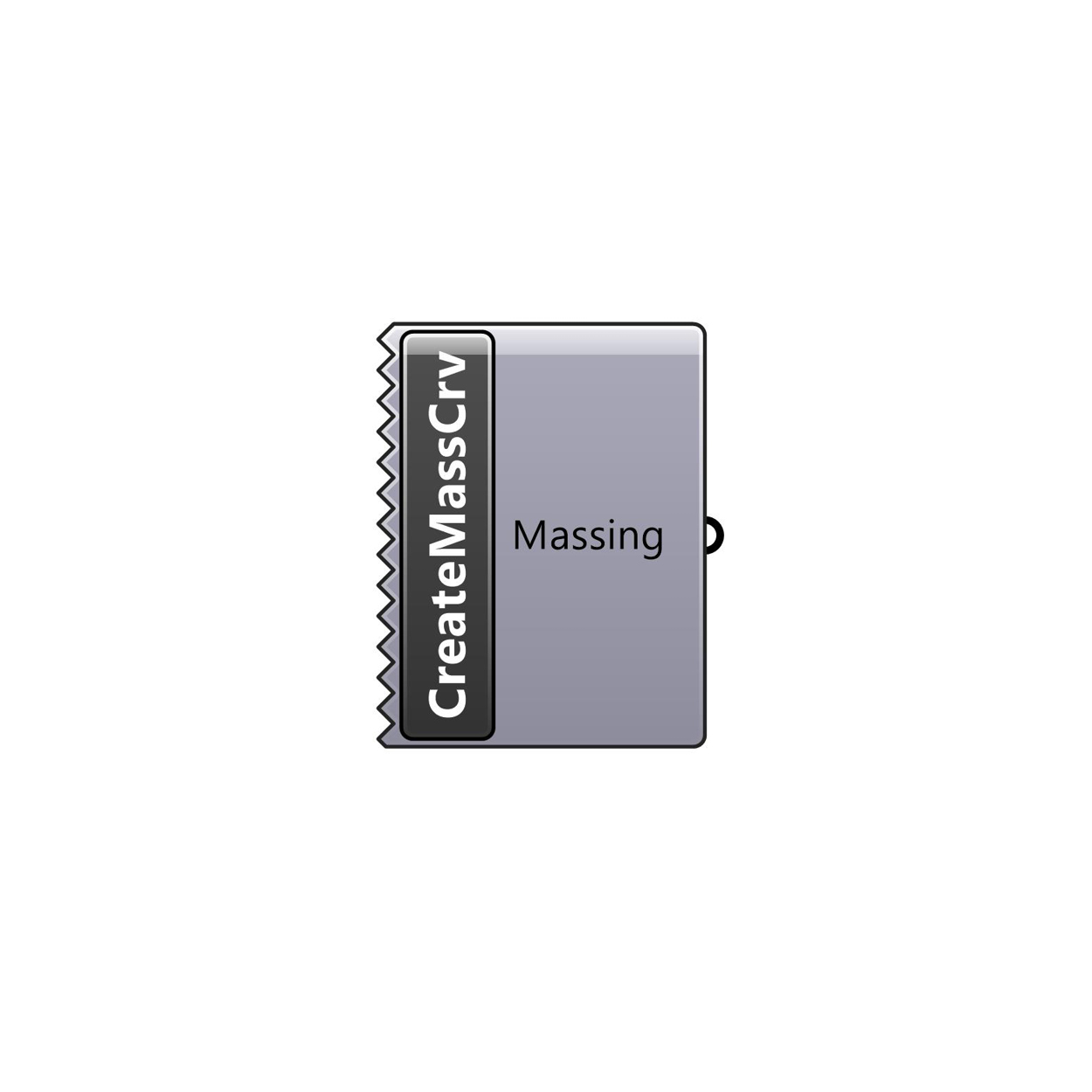### Create Massing from Curve

Creates a MetricMonkey Massing from a closed Rhino polycurve. Polycurve is referenced from the Layer defined in the Rhino Layers card.

Input: N/A

Output: Massing (MetricMonkey Mass).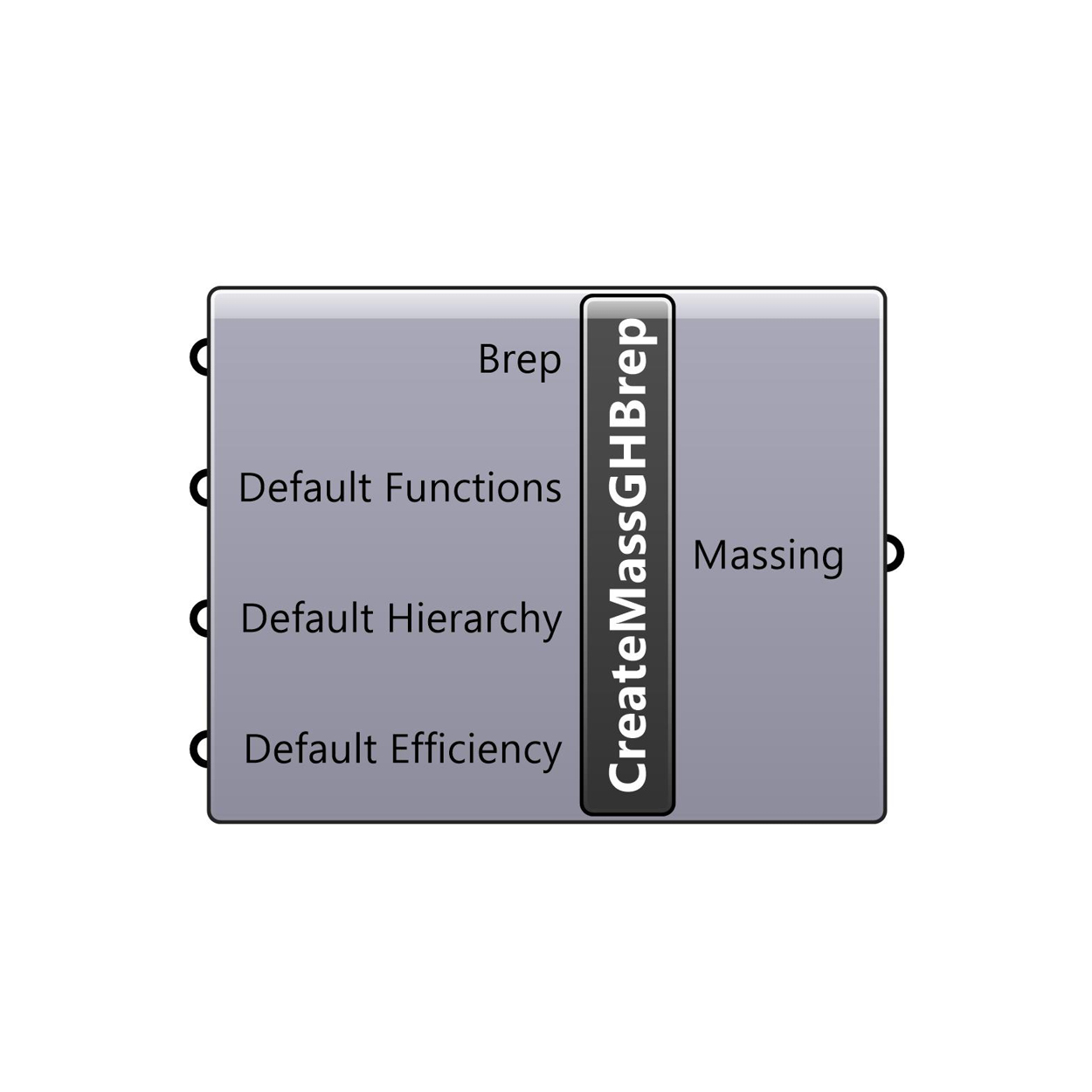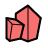### Create Massing from GH Brep

Creates a MetricMonkey Massing from a closed Grasshopper brep.

Input: Brep (Brep), Default Function (MetricMonkey Function), Default Hierarchy (Integer), and Default Efficiency (Integer).

Output: Massing (MetricMonkey Mass).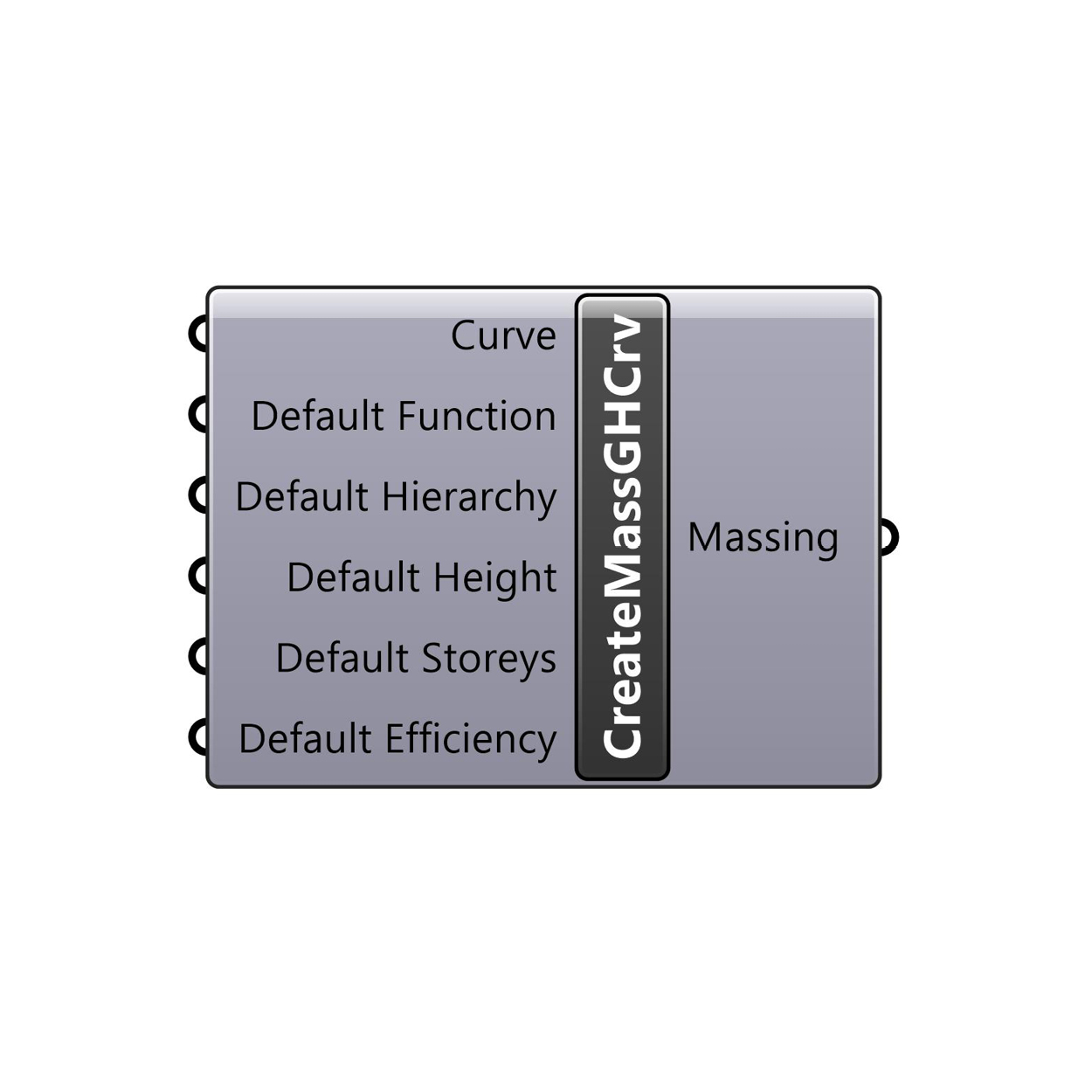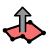### Create Massing from GH Curve

Creates a MetricMonkey Massing from a closed Grasshopper polycurve representing the building footprint.

Input: Curve (Curve), Default Function (MetricMonkey Function), Default Hierarchy (Integer), Default Height (Number), Default Storeys (Integer), and Default Efficiency (Integer)

Output: Massing (MetricMonkey Mass).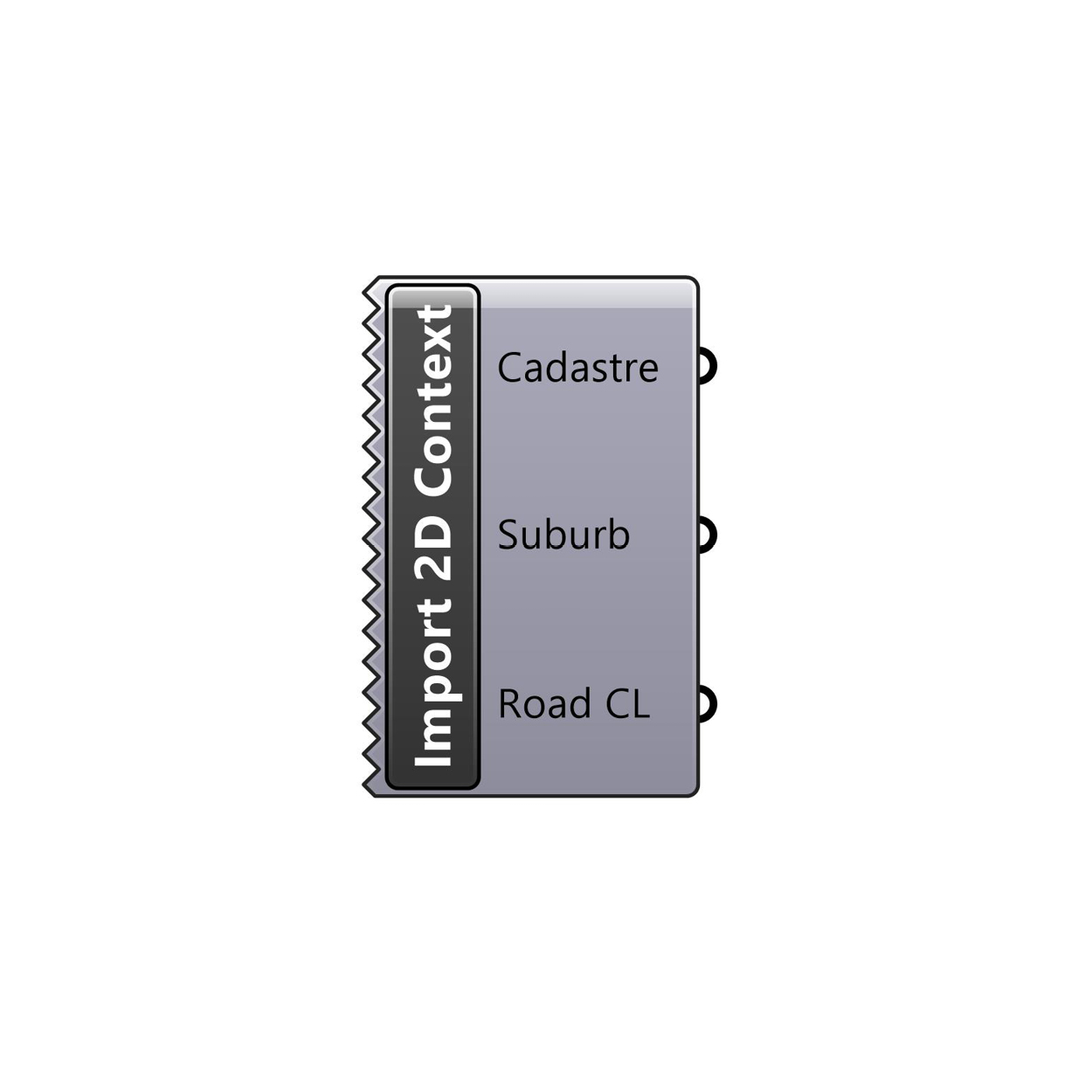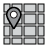### Import 2D Context

Imports 2D context datasets based on the datasets selected in the Import Context card.

Input: N/A### Import 3D Context

Imports 3D context datasets based on the datasets selected in the Import Context card. Use in conjunction with the Simplify Topography component and the Crop Context Buildings component.

Input: N/A

Output: Topography Mesh (Mesh) and Context Buildings (Geometry).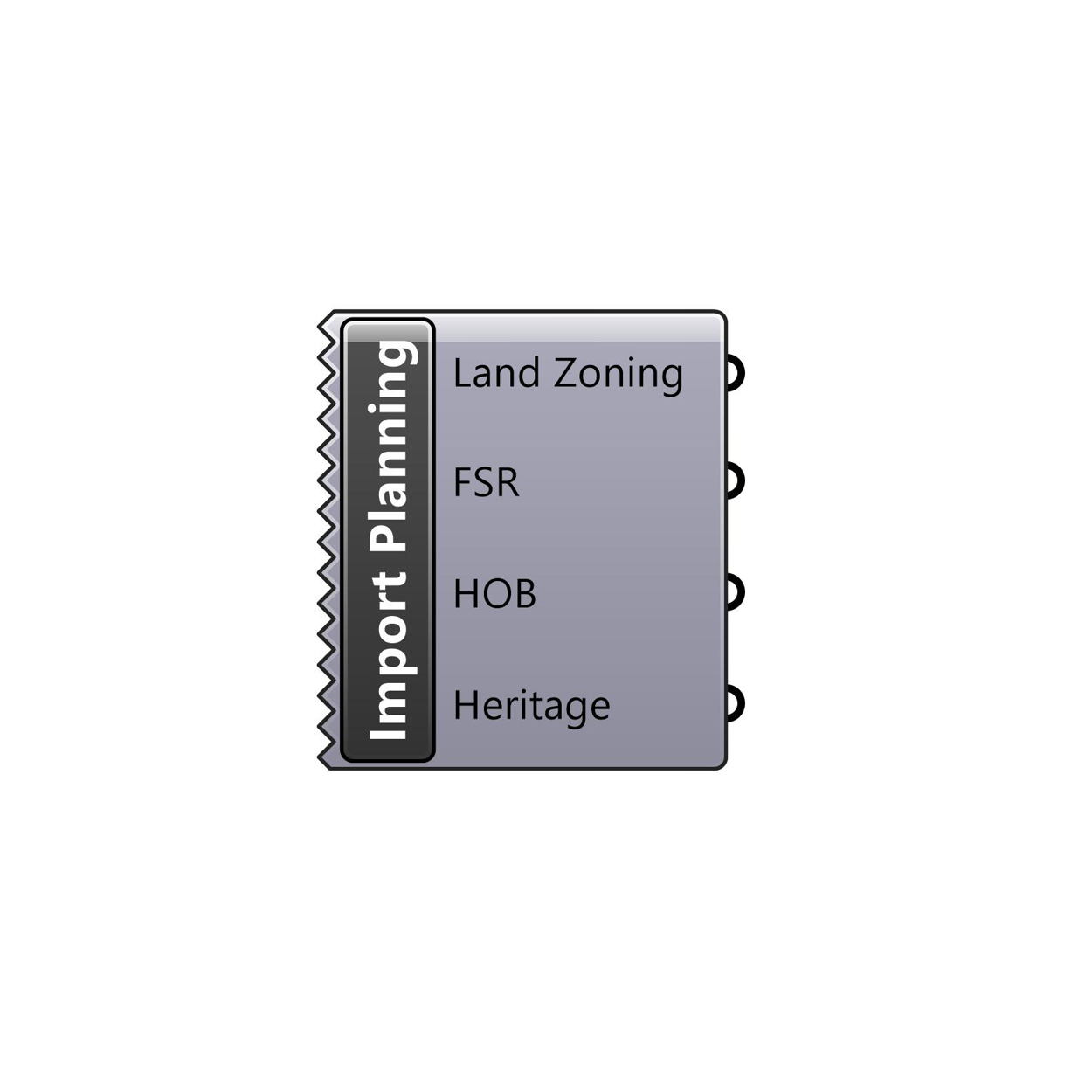### Import Planning

Imports Planning datasets based on the datasets selected in the Import Context card.

Input: N/A

Output: Land Zoning (MetricMonkey Zoning), FSR (MetricMonkey FSR), HOB (MetricMonkey HOB), and Heritage (MetricMonkey Heritage).

## Query

Contains a collection of components to deconstruct MetricMonkey classes.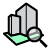### Get Development Properties

Deconstructs a MetricMonkey Development into its constituent properties. Use in conjunction with the Create Development component.

Input: Development (MetricMonkey Development).

Output: Lot Area (Number), FSR (Number), GBA (Number), GFA (Number), Height (Number), MaxRL (Number), and MinRL (Number).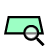### Get Lot Properties

Deconstructs a MetricMonkey Lot into its constituent properties. Use in conjunction with the Create Development component.

Input: Lot (MetricMonkey Lot)

Output: ID (Text), Curves (Curve), Surfaces (Surface), Area (Number), FSR (Number), FSR Allowed (Number), GFA Allowed (Number), Coverage Allowed (Number), Coverage (Number), and Coverage Delta (Number).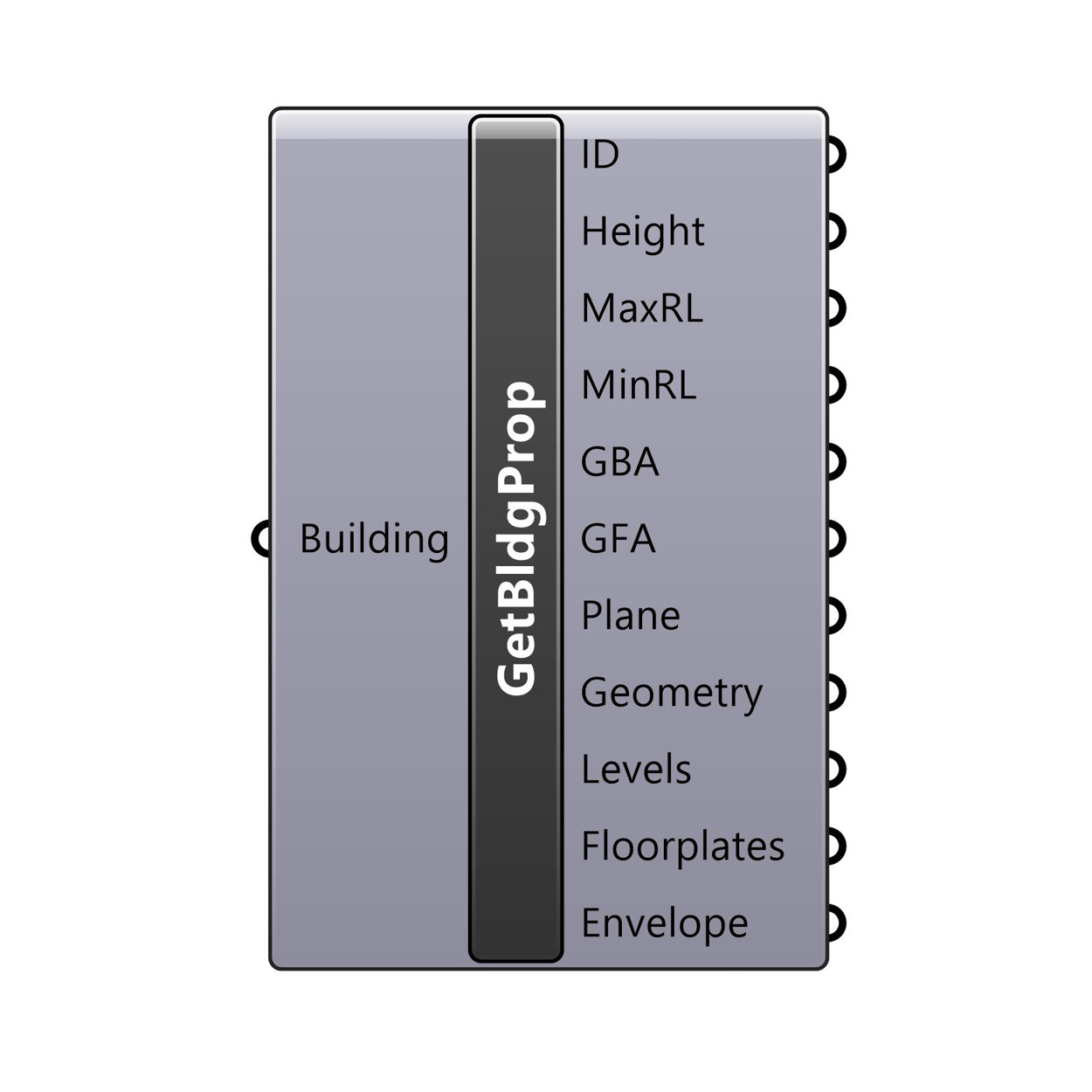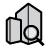### Get Building Properties

Deconstructs a MetricMonkey Building into its constituent properties. Use in conjunction with the Create Development component.

Input: Building (MetricMonkey Building).

Output: ID (Text), Height (Number), MaxRL (Number), MinRL (Number), GBA (Number), GFA (Number), Plane (Plane), Geometry (Geometry), Massing (MetricMonkey Mass), Levels (MetricMonkey Level), Floorplates (MetricMonkey Floorplate), and Envelope (MetricMonkey Envelope).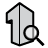### Get Mass Properties

Deconstructs a MetricMonkey building Massing into its constituent properties.

Input: Massing (MetricMonkey Mass).

Output: Height (Number), Storeys (Integer), Hierarchy (Integer), Efficiency Type (Integer), GBA (Number), GFA (Number), Function (MetricMonkey Function), Colour (Colour), Geometry (Geometry), Lot (MetricMonkey Lot), Levels (MetricMonkey Lot), Floorplates (MetricMonkey Floorplate), and Roof (MetricMonkey Roof).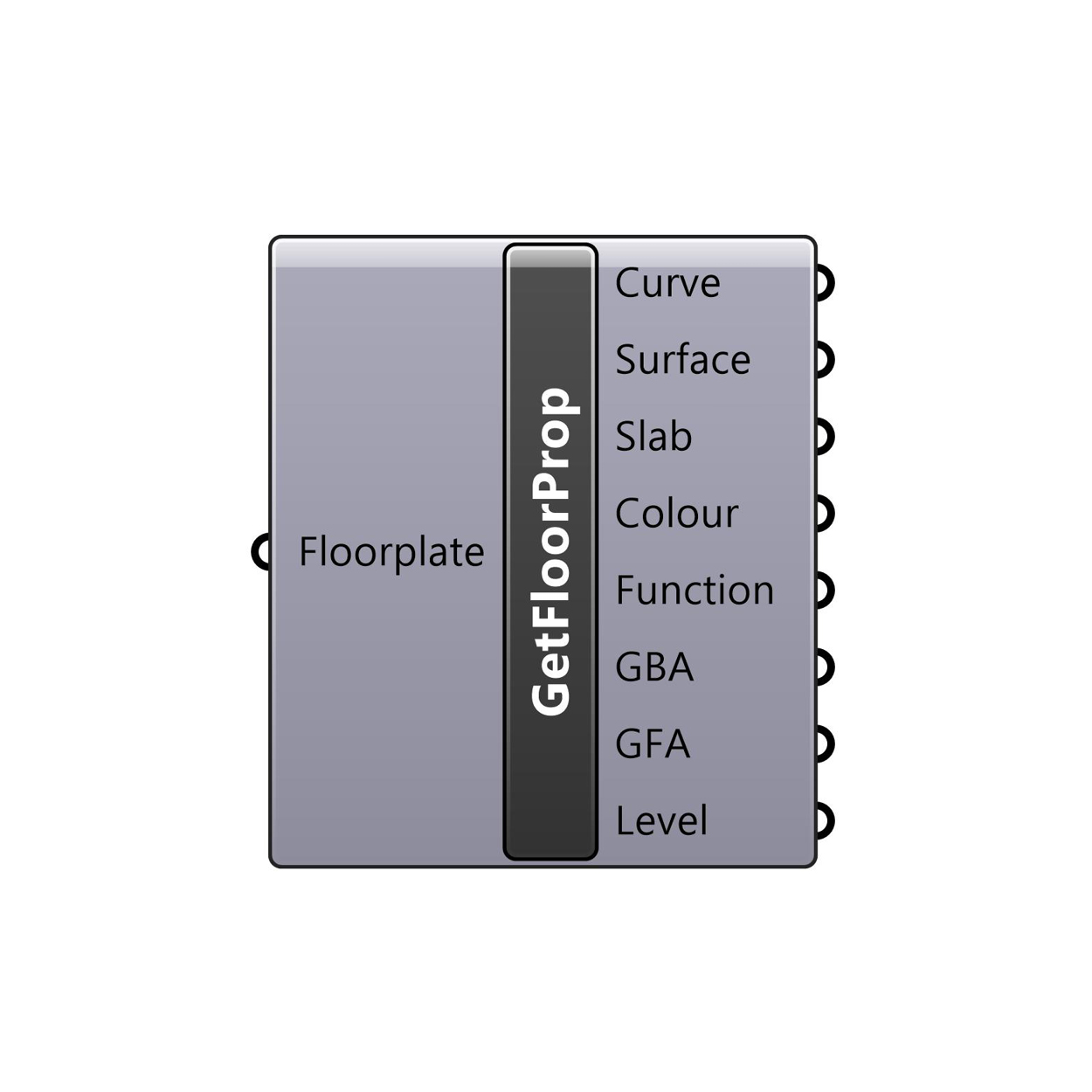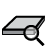### Get Floorplate Properties

Deconstructs a MetricMonkey Floorplate into its constituent properties. Accepts both building and massing floorplates.

Input: Floorplate (MetricMonkey Floorplate).

Output: Curve (Curve), Surface (Surface), Slab (Brep), Colour (Colour), Function (MetricMonkey Function), GBA (Number), GFA (Number), and Level (MetricMonkey Level).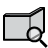### Get Envelope Properties

Deconstructs a MetricMonkey Envelope into its constituent properties. Use in conjunction with the Get Building Properties component (upstream) and the Get Item Properties component (downstream).

Input: Envelope (MetricMonkey Envelope).

Output: Roof Item (MetricMonkey Roof), Facade Item (MetricMonkey Façade), and Base Item (MetricMonkey Base).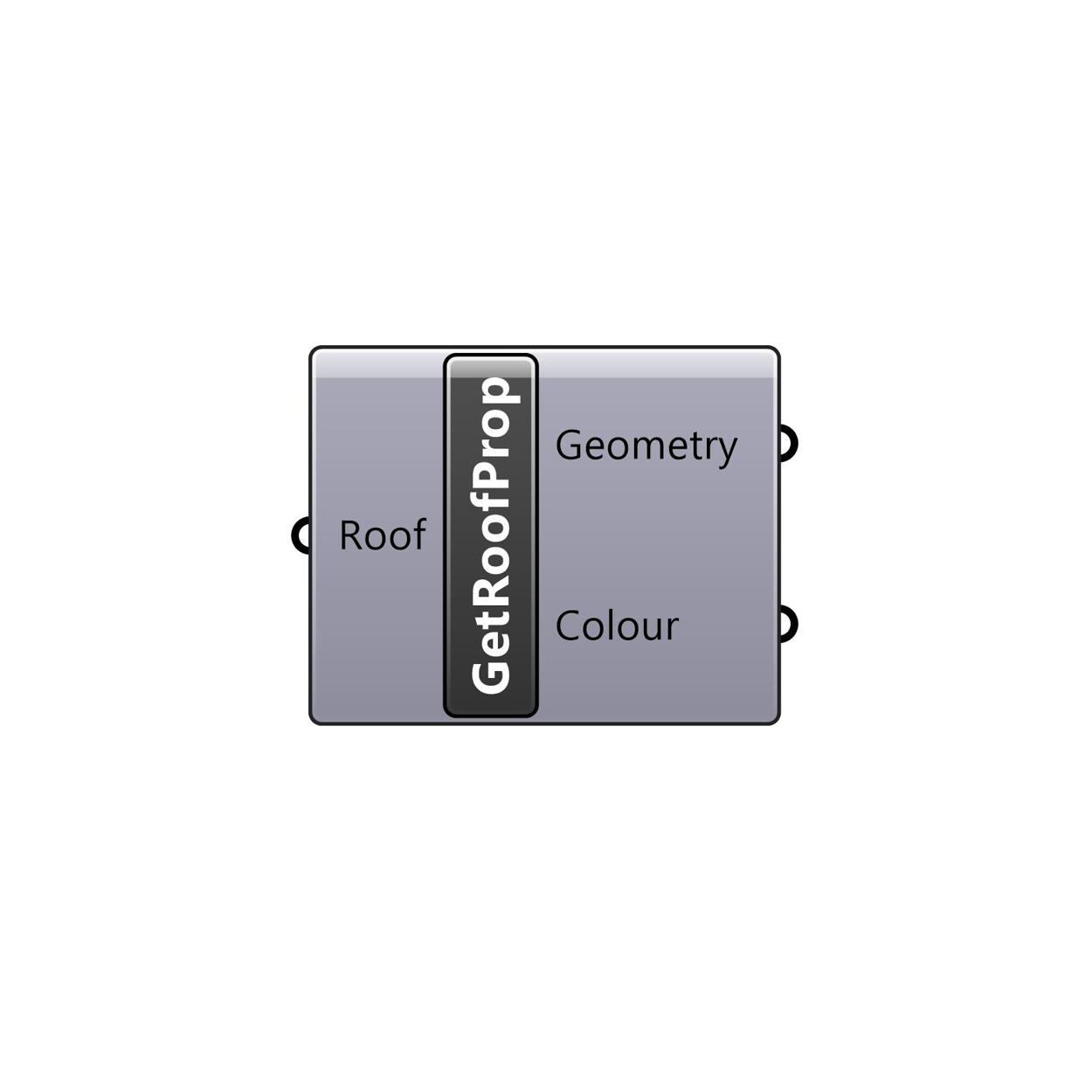### Get Roof Properties

Deconstructs a MetricMonkey Roof into its constituent properties. Use in conjunction with the Get Mass Properties component.

Input: Roof (MetricMonkey Roof).

Output: Geometry (Geometry), and Colour (Colour).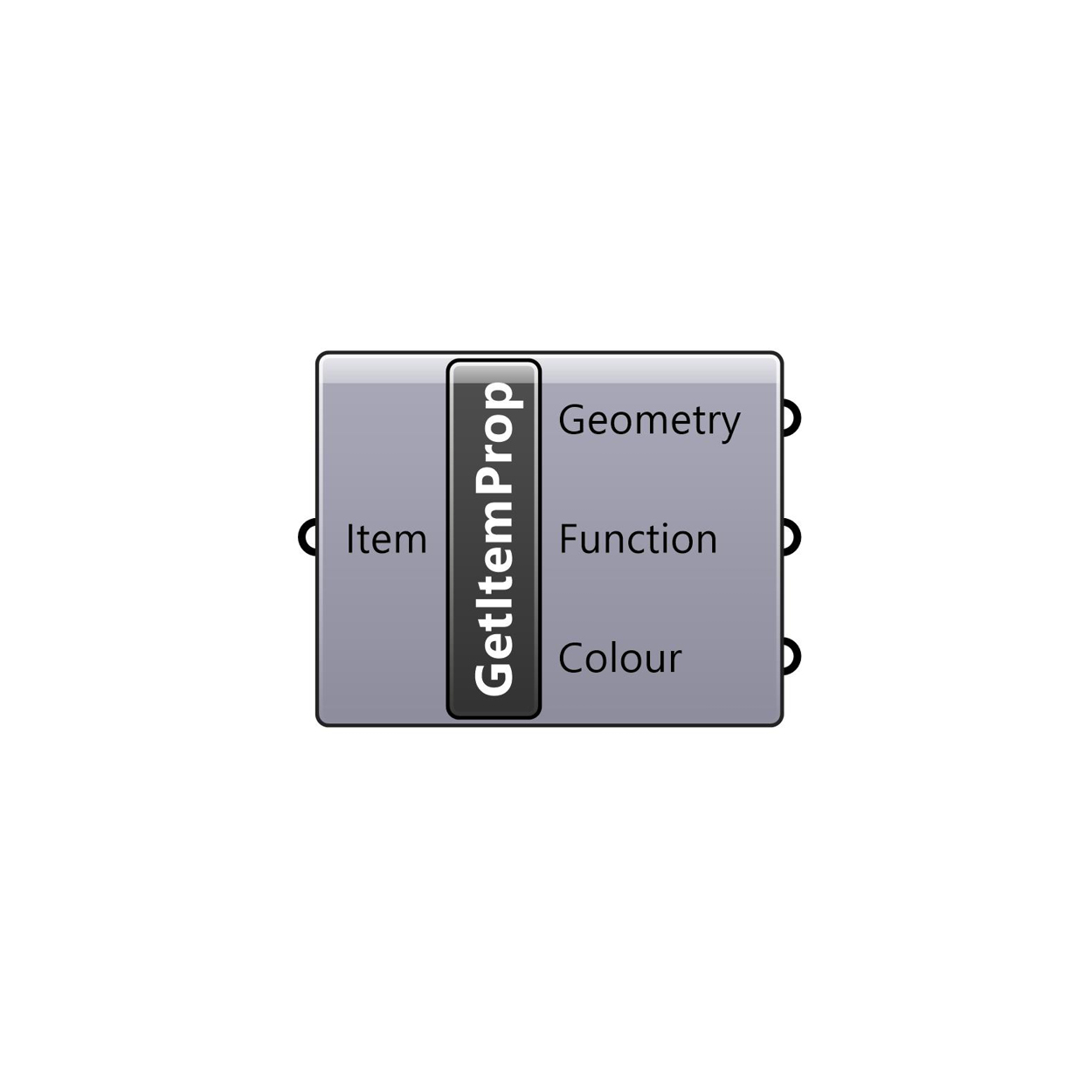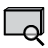### Get Item Properties

Deconstructs a MetricMonkey Item into its constituent properties. Accepts MetricMonkey Roofs, MetricMonkey Façades, and MetricMonkey Base Items. Use in conjunction with the Get Envelope Properties component.

Input: Item (MetricMonkey Item).

Output: Geometry (Geometry), Function (Text), and Colour (Colour).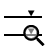### Get Level Properties

Deconstructs a MetricMonkey Level into its constituent properties.

Input: Level (MetricMonkey Level).

Output: ID (Text), Name (Text), Plane (Plane), and Parent (MetricMonkey Building).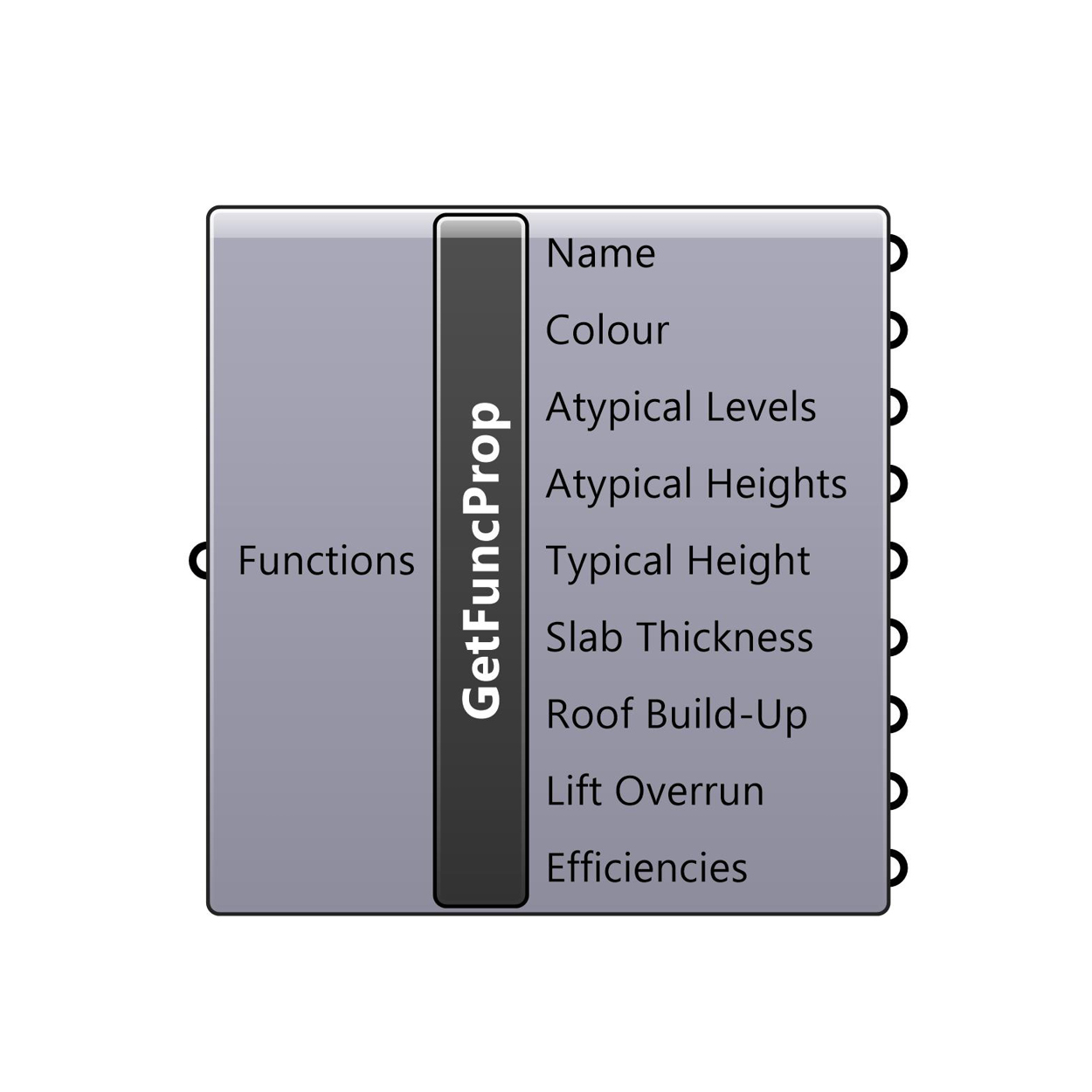### Get Function Properties

Deconstructs a MetricMonkey Function into its constituent properties.

Input: Functions (MetricMonkey Function), and Name (Text).

Output: Name (Text), Colour (Colour), Atypical Levels (MetricMonkey Levels), Atypical Heights (Number), Typical Height (Number), Slab Thickness (Number), Roof Build-Up (Number), Lift Overrun (Number), Efficiencies (Number).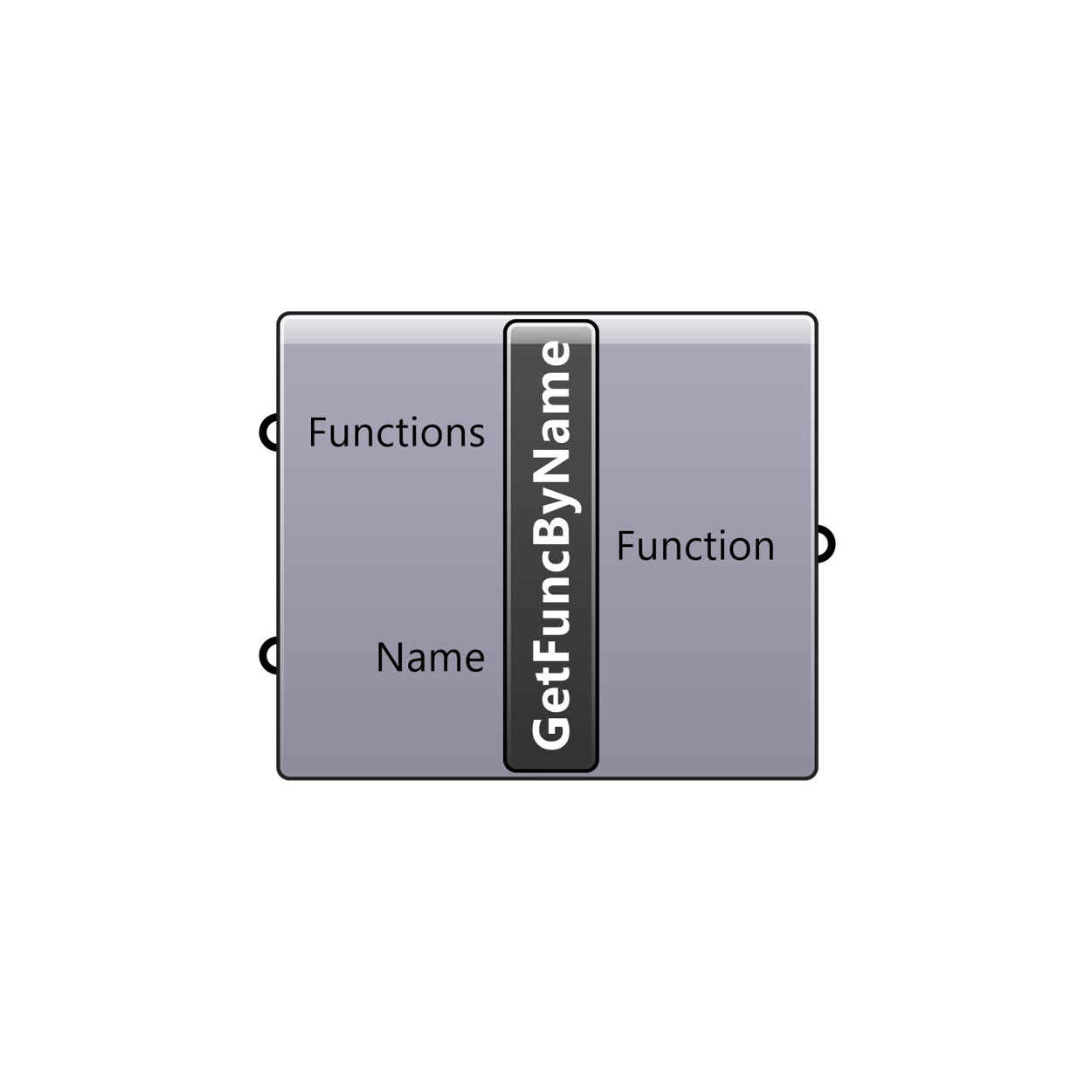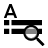### Get Function By Name

Returns a MetricMonkey Function given a name. Use in conjunction with the Function Listener component.

Input: Functions (MetricMonkey Functions), and Name (Text).

Output: Function (MetricMonkey Function).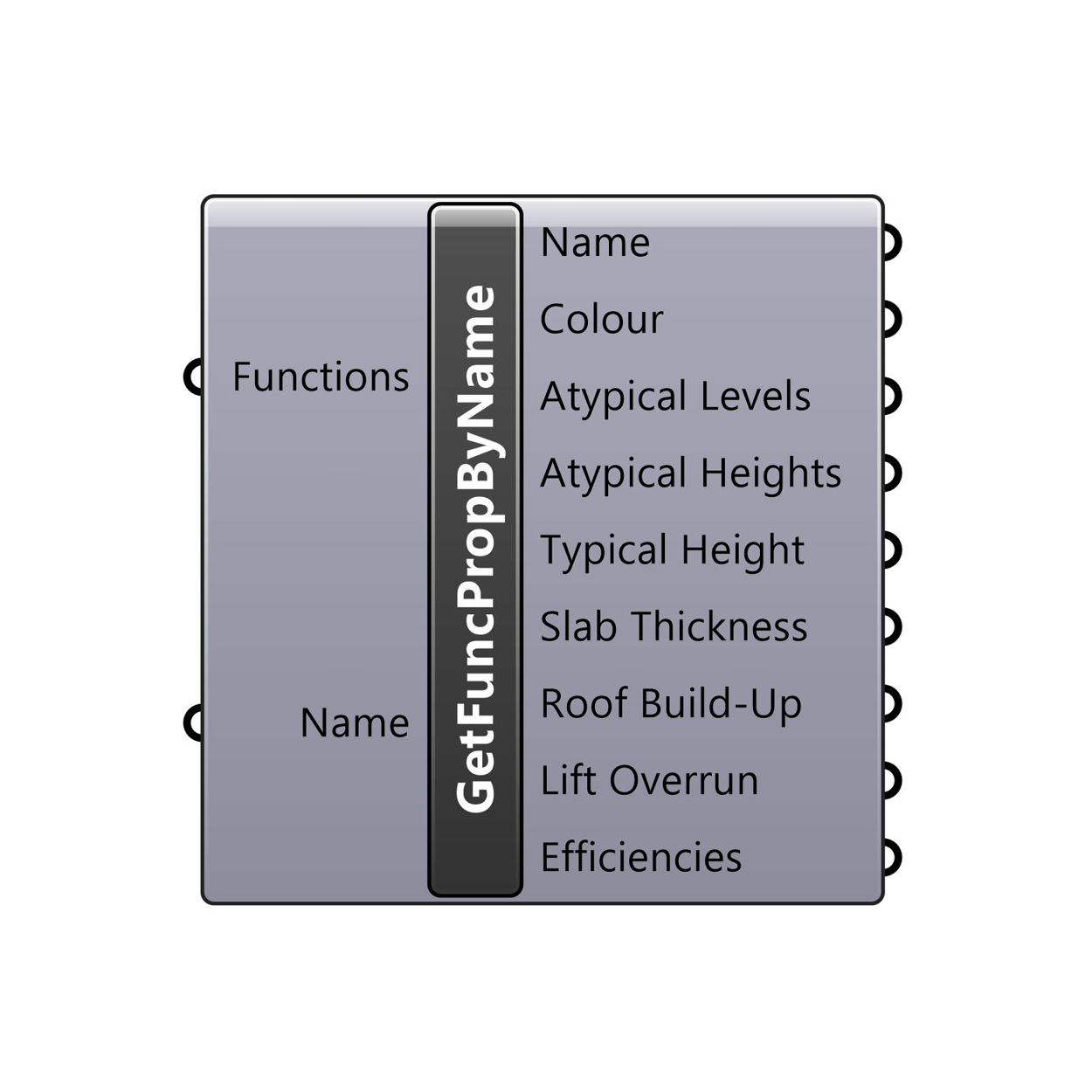### Get Function Properties By Name

Deconstructs a MetricMonkey Function into its constituent properties given a name. Use in conjunction with the Function Listener component.

Input: Functions (MetricMonkey Function), and Name (Text).

Output: Name (Text), Colour (Colour), Atypical Levels (MetricMonkey Levels), Atypical Heights (Number), Typical Height (Number), Slab Thickness (Number), Roof Build-Up (Number), Lift Overrun (Number), Efficiencies (Number).

## Modify### Simplify Topography

Modifies a Topography Mesh by converting it to a Topography Surface using the Sampling Distance to determine the spacing of control points. Use in conjunction with Import 3D Context component.

Input: Topography Mesh (Mesh), and Sampling Distance (Number).

Output: Topography Srf (Surface), and Max Deviation (Number).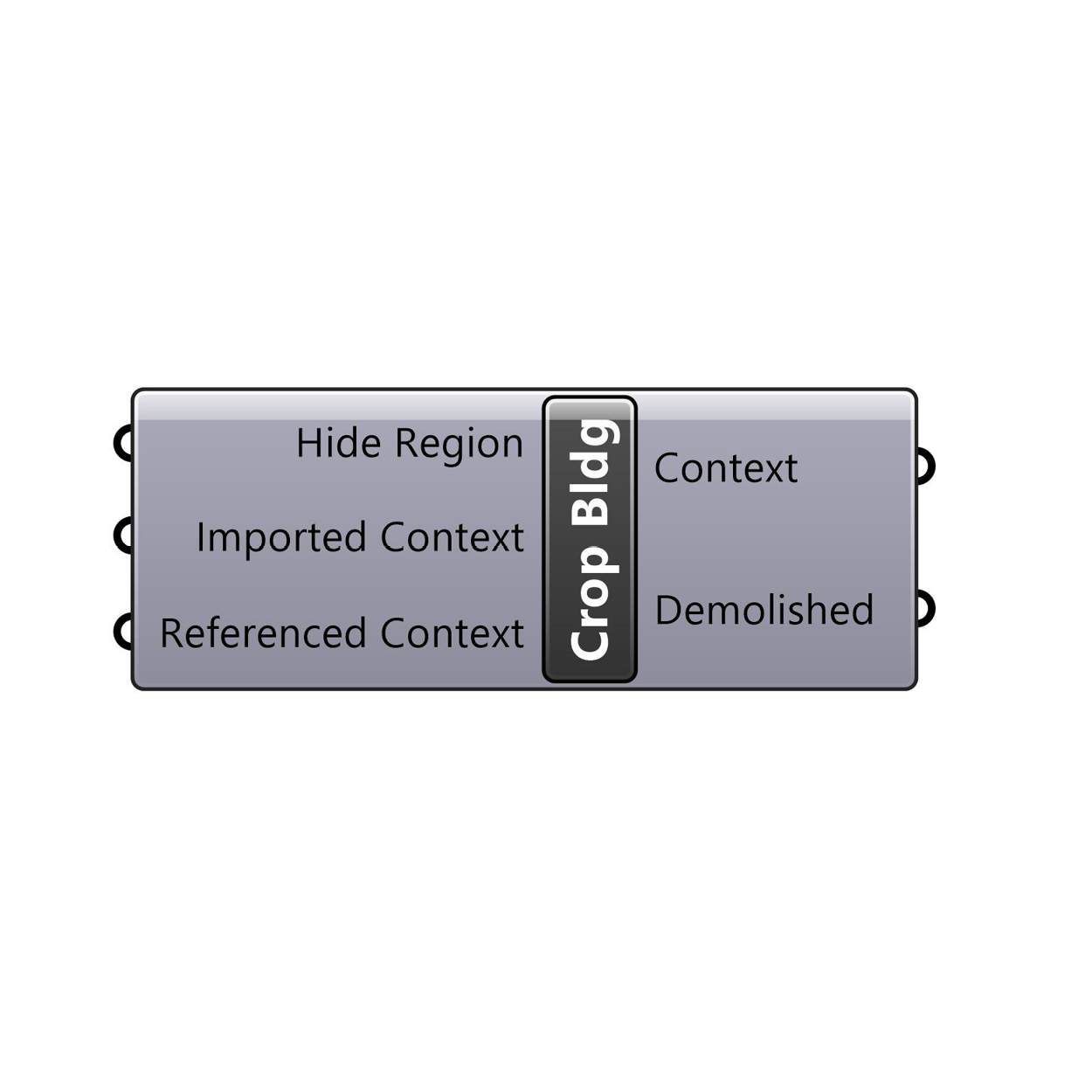### Crop Context Buildings

Separates Context Buildings that are located within the Hide Region. The centroid of the geometry is used to determine containment. Use in conjunction with the Import 3D Context component (upstream).

Input: Hide Region (Polycurve), Imported Context (Geometry), and Referenced Context (Geometry).

Output: Context (Geometry), and Demolished (Geometry).